The Basics

Installing Python on Windows

Below are step-by-step instructions for installing Python 3.9.6 (which is required for the competition):

1. Scroll down “Files” and click on “Windows installer 64 bit” (if you are on a 64 bit machine) or “Windows installer 32 bit” (if you are on a 32 bit machine). If you do not know what version to get, then type in your windows search bar “About your PC”. Under ‘system type’ it should tell you what bit of operating system you have.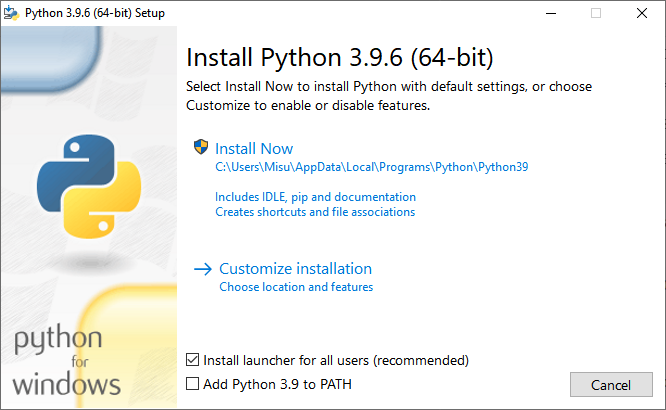4. Tick the “Add Python 3.9 to PATH” checkbox and click “Install Now” (important)

5. Windows will warn you on whether you want to make changes to this device. Click “yes”.

6. If the Python install was successful then you will get this window: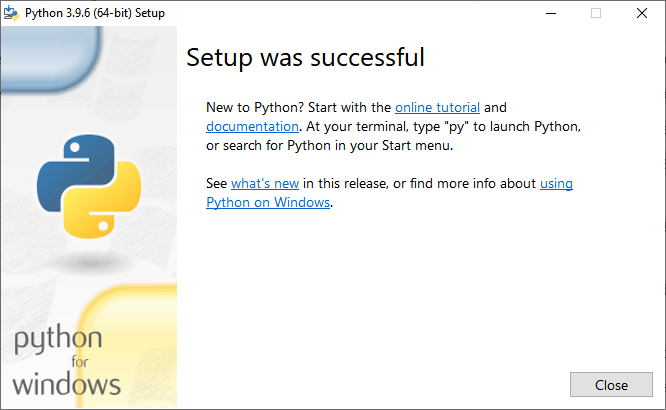Installing Python on Mac

Below are step-by-step instruction for installing Python 3.9.6 (which is required for the competition):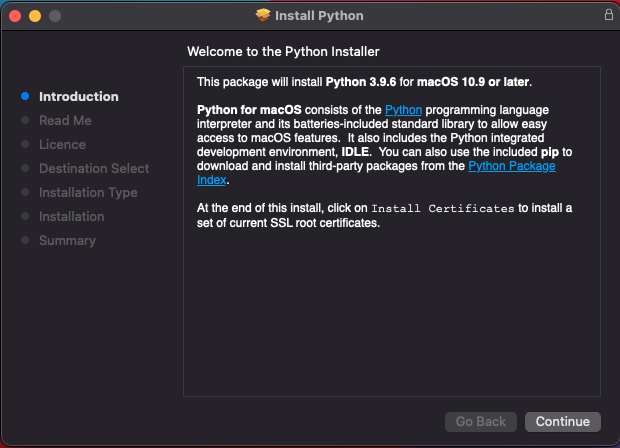3. Follow the rest of the instructions as given by the installer.

A small Introduction

The purpose of this document is to teach/remind you how Python works. Throughout the training booklet, there are links for further resources and websites just in case you are not satisfied with how a particular concept is explained.

Below is a list of websites that have some Python tutorials:

If you are stuck, then you can check if someone else had a similar issue on StackOverflow.

A short overview on how programs work

A program is a list of instructions that are executed, line-by-line by a computer. The instructions are written in a programming language and are converted into binary for the computer to understand.

We are going to be using Python as it is easy to learn and there is an abundance of useful libraries (pre-written code).

To write your own program, you will be using a code editor. Python comes with its own editor called IDLE, but we will use VSCode instead as it has some built-in features that should make collaborative remote programming much easier.

Variables

In a program you often need to store information to use later; to do this you would use a variable.

Variables are called that because the value stored in one can change, or vary. Some languages have constants whose values can’t change but these don’t exist in Python.

To assign a value to a variable in Python we specify a name for the variable, followed by the =sign, followed by the value e.g:

name = "Mary"
age = 17

A variable could store many types of data including numbers, text, collections of numbers or text, entire robots and much more.

Here are some of the built-in types:

Name of type Example
String "Hello World"
Integer 6
Float 4.2
Boolean True
List [1, 2, 3, 4, 5, 6]
Dictionary {"a": 1, "b": 2}

In Python you don’t need to tell the computer what type your variable is, the computer will just work it out based on the value you assign to it. It’s generally frowned upon to change a variable’s value to a different type, although Python does let you do it.

The print statement displays what it’s given in the output window. This is useful for debugging as you can use it to see what value a variable is storing. For example:

print("Hello world")

Would output the following in the console:

Hello world

We can also print variables. Here, variable a stores the number 4.2:

>>> a = 4.2
>>> print(a)
4.2

Input Statement

input() is the opposite of print(). It lets you type something in and get it as a variable. Here are a few examples:

>>> a = input()
Hello
>>> print(a)
Hello

As you can see, the program waits for you to give an input which is Hello in this example. When you print a it outputs the string Hello - which is what was inputted.

input() always returns a string, so If you want to input numbers (integers or floats) then you will need to convert the input to the appropriate variable type using the int() or float() functions.

a = int(input())

While you won’t be able to use input() in the competition (the robots don’t have keyboards), it can be quite useful for practising or for testing ideas.

Commenting

Making the intent of your code clear is very important, even more so when working in a team. Use comments to explain why the code is behaving a certain way without affecting the program flow. Any text after a ‘#’ will be ignored e.g:

# A comment on its own line
favourite_insect = "Bee"  # Bees are cool. (Comment on same line as code)

Data Types

Storing text - Strings

Strings are used to store a bunch of characters. To create a string, put speech marks ("") around the text that you want to be in the string. For example:

>>> a = "Foo"
>>> a
'Foo'
>>> "abc" + "def"  # Concatenate 2 strings together
'abcdef'
>>> str(42)  # Convert something else to a string
'42'

Storing numbers - Integers and decimals

As the name implies, integer variables store integers (whole numbers). In Python decimals (known as floats) and integers can be used interchangeably and will work with each other without you having to think about it.

bees = 100
honey = 0.3
total_honey = honey * bees  # evaluates to 30

Operations involving numbers are covered in the Doing Maths in Python section.

Storing Collections - Lists

Lists are a bunch of values under one variable. They are defined by putting the values within square brackets and separating the variables with commas, like this:

a  =  [1, 2, 3, 4, 5, 6, 7]

Get certain items from a list using the list index. The index starts at 0:

a  # Evaluates to 1
a[-1]  # Evaluates to 7 - negative numbers count backwards from the end

As it so happens, strings are lists of characters! You can use list operations on them too:

alpha = "abcdefg"
letter = alpha  # letter is "e"
cabbage = alpha + alpha + alpha*2 + alpha + alpha + alpha

True / False logic - Booleans

Boolean variables can only be True or False. Booleans are used for conditions in if statements, while loops and for loops. These will come up later.

You can use boolean operators to make complex decisions based on lots of variables.

Symbol/Operator Description Example that would be True Example that would be False
== Equals 1 == 1 1 == 2
!= Does not equal 1 != 2 1 != 1
> Greater Than 4 > 2 3 > 4
< Less Than 2 < 4 4 > 1
>= Greater Than or Equal To 4 >= 4 4 >= 6
<= Less Than or Equal To 2 <= 2 7 >= 1
and Logical ‘and’ operator True and True False and False
or Logical ‘or’ operator True or False False or False
not Logical ‘not’ operator not False not True

Using Logic

If Statements

If statements let you execute code depending on whether the condition evaluates to true, e.g:

name = "mary"
if len(name) > 3:
print("Your name isn't all that short")

You can add an else statement following your if statement to execute code if the condition is false, e.g:

name = "mary"
if len(name) > 3:
print("Your name isn't all that short")
else:
print("Wow that's a short name")

If you want to chain if statements together you can use the elif statement like so:

name = "mary"
if len(name) > 3:
print("Your name isn't all that short")
elif len(name) > 10:
print("Wow that's a long name")
else:
print("Wow that's a short name")

While Loops

A while loop will continue executing the code within it while the condition is true, e.g:

while True:
print("I know a song that will get on your nerves")
print("I know a song that will get on your nerves")
print("And this is how it goes")

In order to not get ‘stuck’ in a while loop you should make sure that you change the condition within the loop:

number_of_sheep = 0
while number_of_sheep < 10:
print("Hope I sleep soon")
number_of_sheep += 1
print("zzzzzzzzz")

For Loops

An for loop and an in statement lets you loop through each item in a list, e.g:

tasty_types_of_cheese = ["Cheddar", "Camembert", "Gouda", "Brie"]
for cheese in tasty_types_of_cheese:
print(cheese + " is tasty")

Indentation

Indentation is important in Python. For code to be counted as part of a loop, if statement or function it has to be indented properly. The standard indent size is 4 spaces. Pressing ‘tab’ in VSCode will automatically indent the configured amount.

tasty_types_of_cheese = ["Cheddar", "Camembert", "Gouda", "Brie"]
for cheese in tasty_types_of_cheese:
print(cheese + " is tasty")  # Part of the loop
print("mmm yum")  # Not indented so not part of the loop

• If Statements
• While Loops
• For Loops

Doing Maths in Python

Basic Operators

Maths in Python is very similar to the way we do maths in a calculator. The order of operations follows BIDMAS (Brackets, Indices, Division, Multiplication, Addition and Subtraction). We would recommend using a liberal amount of brackets to ensure that the correct order of operations is carried out.

You can also use variables when doing mathematical operations, however they need to be floats or integers. Mathematical operations won’t always work on strings or booleans.

Below is a list of mathematical operators that you can use:

Operator Name in Python Operator in mathematical form
Add a + b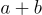Subtract a - b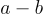Divide a / b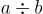Multiply a * b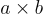Powers a ** b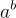Using the math module

The math module has several mathematical functions that could be useful for robot development (e.g trigonometry functions). To access this module, it must be imported.

>>> import math
>>> math.factorial(5)
120
>>> math.cos(0)
1.0

Debugging (and better print statements)

Sometimes you might come across issues with your code that you may need to debug. The print() statement comes in handy in these situations. You might find it useful to insert print() statements at various points in your code to track the values of variables:

x = 40
print("initialised x as 40")
x = x + 2
print("x is now:", x)

f-strings

For inserting values into strings we recommend f-strings. f-strings look like normal strings, but with an f in front like this: f"This is an f-string". Unlike normal strings, f-strings let you insert values into them inside curly brackets {} like this:

var_age = 20
print(f"my age is {var_age}"))  # Prints "my age is 20"

You can put any valid value in the curly brackets:

f"{["Mammal", "Reptile", "Fish", "Amphibian", "Bird"]} is the word"

Functions

Functions are named blocks of reusable code. They’re useful for making your code neater and easier to read, which is important as you don’t have that much time to debug errors. Using functions reduces the amount of repeated code in your program. Make them like this:

def function_name():
print("This function will print this string")

This function prints "This function will print this string" every time it’s called.

>>> function_name()
This function will print this string

Functions can take in extra data called parameters. These work like variables that you can only access from within the function, and their values get set when the function gets called.

def turn_right(angle, speed):
print(f"set the left motor to {speed}")
print(f"set the right motor to {-speed}")
print(f"wait {angle / 100} seconds")
print("set the left motor to 0")
print("set the right motor to 0")

Here’s what happens when you call turn_right:

>>> turn_right(45, 0.7)
set the left motor to 0.7
set the right motor to -0.7
wait 0.45 seconds
set the left motor to 0
set the right motor to 0

Functions can also return values, which can then be stored in variables, passed to other functions or even just ignored. Here’s a function which returns a value:

import math
return degrees * math.pi / 180

And here’s that function in action:

6.283185307179586

Scope of a variable

Not all variables are accessible in every part of the code. Where a variable can be accessed from is called its scope. If the variable is defined in a function, then you can only use that variable inside the same function. Variables defined outside functions can be used anywhere.

If you want a variable in a function to be accessible everywhere, write global in front of its name when you define it (e.g global myVar = 2). You shouldn’t usually need to do this, though.